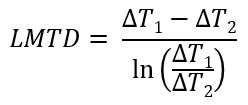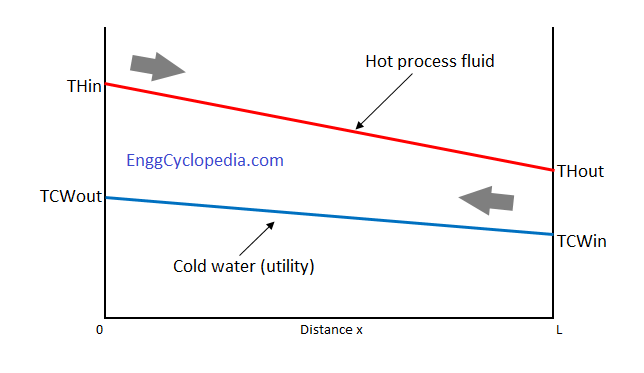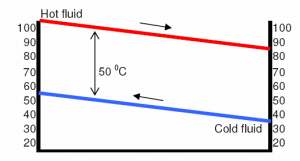# Logarithmic Mean Temperature Difference (LMTD) calculation

The Log Mean Temperature Difference (LMTD) is a mathematical formula used in the design of heat exchangers to calculate the temperature difference between the hot and cold fluid streams at different points in the exchanger. The LMTD is used to determine the heat transfer rate, which in turn is used to design the heat exchanger size, type, and cost.

## LMTD Equation

As per the definition and equation for Log Mean Temperature Difference (LMTD),ΔT1 → the temperature difference between hot and cold fluids at one end of the heat exchanger
ΔT2 → the temperature difference between hot and cold fluids at the other end of the heat exchanger## Why Is LMTD Important?

Efficiency is the name of the game when it comes to heat exchangers, and LMTD is central to achieving it. Here's why it matters:

1. Design and Sizing: LMTD calculation plays a fundamental role in designing heat exchangers. Engineers use it to determine the size, configuration, and heat transfer area required for a specific application. An optimal LMTD ensures efficient heat exchange.

2. Performance Evaluation: In operation, heat exchangers might not always maintain a constant temperature difference. LMTD helps evaluate how well a heat exchanger performs under real-world conditions. If the actual LMTD differs from the calculated one, adjustments can be made to improve performance.

3. Energy Efficiency: The more efficient the heat exchange, the less energy is required to achieve the desired temperature changes. This translates into energy savings, reduced operational costs, and a smaller environmental footprint.

4. Process Optimization: LMTD is vital in industries where temperature control is crucial, such as chemical processing, pharmaceuticals or food manufacturing. Accurate temperature management ensures consistent product quality and safety.

## LMTD or Log Mean Temperature Difference Calculation

#### Problem Statement:

Calculate the (LMTD) log mean temperature difference for the following shell and tube heat exchanger for two possible arrangements.

(i) counter-current and (ii) co-current flow patterns

Hot fluid in temperature TH1 = 100 0C
Hot fluid out temperature TH2 = 90 0C
Cold fluid out temperature TC2 = 50 0C
Cold fluid in temperature TC1 = 30 0C

#### Solution

(i) For counter current heat exchanger,
ΔT1 = TH1 - TC2 = 100 - 50 = 500C (At one end hot fluid enters and cold fluid exits.)
ΔT2 = TH2 - TC1 = 90 - 30 = 600C

(At the other end cold fluid enters and hot fluid exits.)

by definition given above, LMTD for counter current flow = (60-50) / ln(60/50) = 10 / 0.1823 = 54.850C.
This can be also verified quickly in EnggCyclopedia's LMTD calculator.

(ii) For co-current heat exchanger,
ΔT1 = TH1 - TC1 = 100 - 30 = 700C (At first end hot and cold fluids enter the heat exchanger.)
ΔT2 = TH2 - TC2 = 90 - 50 = 400C

(At the other end hot and cold fluids exit the heat exchanger.)
by definition given above, LMTD for counter current flow = (70-40) / ln(70/40) = 30 / 0.5596 = 53.610C. This can be also verified quickly in EnggCyclopedia's LMTD calculator.

#### Remarks

It can be readily noticed that for co-current heat exchanger the logarithmic mean temperature difference is lower compared to counter-current heat exchanger. This suggests that for same fluids, to achieve the same heat transfer, a co-current heat exchanger always requires more heat transfer area than the counter-current type exchanger.

In this example, a simple heat exchanger with single shell pass and single tube pass is considered. For multiple shell and tube passes, the LMTD calculated has to be multiplied by a correction factor to account for geometric changes. EnggCyclopedia's LMTD correction factor calculator can be readily used for this correction factor calculation.

## LMTD Calculation When The Formula Does Not Work

#### Problem statement:

Calculate (LMTD) log mean temperature difference for the following shell and tube heat exchanger with counter-current flow.

Hot fluid in temperature TH1 = 100 0C
Hot fluid out temperature TH2 = 80 0C
Cold fluid out temperature TC2 = 50 0C
Cold fluid in temperature TC1 = 30 0C

As per the definition and equation for Log Mean Temperature Difference (LMTD),

LMTD = (TH1-TC2 – (TH2-TC1 / log ( (TH1-TC1) / (TH2-TC2) ).. (for counter-current flow)

= (100-50) – (80-30) / log (50/50)

= 0 /0 → Indeterminate!!?

When you are working on online engineering degrees this is sometimes the kind of question you may have. Here is the solution.Plainly using the LMTD formula is clearly misleading in this case. It can be observed that the temperature difference between hot and old media is 50 0C at both ends of the exchanger. Following figure for temperature profile of the counter-current flow, suggests that the temperature difference is always constant and equal to 50 0C. Hence the mean temperature difference should also be equal to 50 0C and clearly is not indeterminate.

## LMTD Calculation FAQs

1. Can LMTD be applied to all types of heat exchangers?

LMTD is widely applicable to various heat exchanger types, such as shell-and-tube, plate, and finned tube heat exchangers. Different exchanger designs may result in different LMTD profiles, but the concept remains universally relevant.

2. Where can I find LMTD calculations and examples for my specific application?

EnggCyclopedia's LMTD calculator can be used for quickly calculating LMTD for different heat exchangers. And this post explains how you manually do the calculation when you know the inlet / outlet temperature values for hot and cold sides.

3. What are some practical applications of LMTD?

LMTD is applied in a wide range of industries and processes, including:

• Heating, Ventilation, and Air Conditioning (HVAC): LMTD is used to design and evaluate heat exchangers in HVAC systems for efficient climate control.
• Chemical and Petrochemical Processes: In these industries, LMTD plays a critical role in optimizing heat exchangers for various reactions and operations.
• Power Generation: LMTD helps enhance the efficiency of heat exchangers used in power plants to convert thermal energy into electricity.
• Food and Beverage Production: It ensures consistent temperatures during food processing and pasteurization.

4. What happens if LMTD is not taken into account in heat exchanger design?

Failure to consider LMTD in heat exchanger design can lead to inefficiencies and suboptimal performance. It may result in oversized or undersized heat exchangers, leading to increased energy consumption, operational costs, or even system failure.

5. How does LMTD vary in different types of heat exchangers?

Different types of heat exchangers, such as shell and tube, plate, or finned tube heat exchangers, have distinct LMTD profiles. The choice of heat exchanger type depends on the specific application and heat transfer requirements. Some configurations are more effective for certain processes than others.

6. Can LMTD be used in industries other than HVAC or chemical processing?

Absolutely. LMTD is a universal concept applicable in a wide range of industries, including power generation, food and beverage processing, pharmaceuticals, and more. Any industry that involves heat transfer processes can benefit from understanding and applying LMTD.